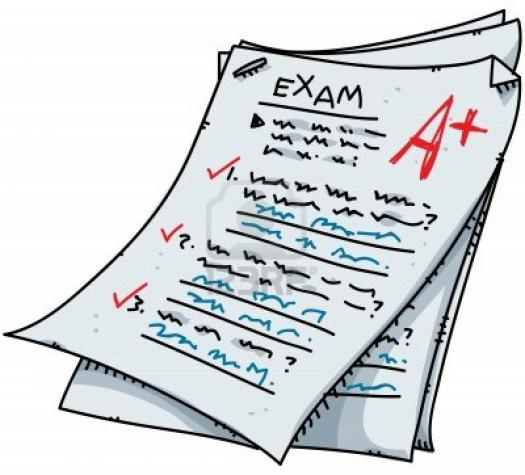# Practice Paper For SBI Clerk 2014

20 Questions | Attempts: 185
ShareSettingsSBI Clerk exam practice below is a good way to practice on the basic Mathematics associated with the profession. How good are you with the topic? There is only one way to find out. All the best.

• 1.
Find the value of the following: 2log25+8log16-5log32+3log5
• A.

16

• B.

25

• C.

7

• D.

32

• 2.
Find the value of x in the following: log5+log(2x-1)-log7=log(x+1)
• A.

4

• B.

5

• C.

9

• D.

2

• 3.
Solve the following for x: 11x+7-7x+9=x+67
• A.

34

• B.

17

• C.

20

• D.

62

• 4.
The average of two numbers is 52. Find the numbers, if the difference of the numbers is 12.
• A.

66,32

• B.

43,52

• C.

28,32

• D.

58,46

• 5.
The age of a father is six times the age of his son. Twenty years from now, father's age will be twice as old as son's age. What is the father's present age?(in years)
• A.

30

• B.

25

• C.

42

• D.

35

• 6.
Twenty years from now, Sagar will be six times as old as he was twenty years ago. What is the present age of Sagar?
• A.

20 years

• B.

28 years

• C.

34 years

• D.

26 years

• 7.
The sum of three consecutive odd numbers 33. Find the sum of the squares of the numbers.
• A.

371

• B.

257

• C.

362

• D.

410

• 8.
Solve the following pairs of equations for x and y. 3x-2y-5=0 and 5y-4x+2=0
• A.

5,4

• B.

2,1

• C.

4,2

• D.

3,2

• 9.
The ratio of ages of A and B is 7:9. If B is twelve years older than A, What are the respective ages of A and B in years?
• A.

42,54

• B.

35,49

• C.

28,63

• D.

32,47

• 10.
If a:b=2:3, b:c=3:4, c:d=8:5, e:d=7:10, and f:e=15:14 then find f:a.
• A.

15:16

• B.

3:18

• C.

5:17

• D.

11:21

• 11.
The ratio of the ages of A, B and C is 7:5:4. If C's age is 32 years, find the sum of ages of A, B and C (in years).
• A.

128

• B.

120

• C.

153

• D.

98

• 12.
If b:a=3:1, d:c=3:2, e:d=13:12, f:e=1:1 and b:c=3:8, then find a:e:f.
• A.

1:2:3

• B.

13:2:11

• C.

1:2:3

• D.

1:13:13

• 13.
A's salary is 30% more than B's salary. B's salary is 20% less than C's salary. What percentage of C's salary is A's salary?
• A.

104%

• B.

98%

• C.

120%

• D.

80%

• 14.
An article was marked 35% above the cost. If a profit of 8% is made by selling the article, what is the discount percent offered on the article?
• A.

25%

• B.

20%

• C.

15%

• D.

40%

• 15.
Sam, Joy and Ben invest Rs.80,000, Rs.64,000 and Rs.48,000 respectively in a partnership firm. If at the end of one year, Ben earns a profit of Rs.10,800, then what is the total profit earned by the firm?
• A.

Rs.38,400

• B.

Rs.27,300

• C.

Rs.43,200

• D.

Rs.52,000

• 16.
An article is 20% over the cost. A discount of 8% is offered on the marked price of the article. What is profit percentage in selling the article?
• A.

14%

• B.

10.4%

• C.

9.5%

• D.

17%

• 17.
Rs.20,000 at 10% p.a.  Compound interest for two years, Compounded annually?
• A.

Rs.24,000

• B.

Rs.22,000

• C.

Rs.26,000

• D.

Rs.24,200

• 18.
A man borrows Rs.80,000 at 15% p.a. Simple interest. He repays Rs.48,000 at the end of first year and the balance at the end of second year.  What amount did he repay at the end of the second year?
• A.

Rs.48,800

• B.

Rs.45,000

• C.

Rs.52,000

• D.

Rs.41,200

• 19.
The average age of a group of four men whose ages are in the ratio 4:5:6:7 is 44 years. What is the age of the eldest person in this group?
• A.

52 years

• B.

56 years

• C.

64 years

• D.

48 years

• 20.
What quantity of wheat at Rs.10 per kg should be mixed with 48 k gs of wheat at Rs.6 per kg and 30 k gs of wheat at Rs.8 per kg, such that a profit of 25% is earned on selling the mixture at Rs.10 per kg?
• A.

48 kg

• B.

40 kg

• C.

54 kg

• D.

39 kg

## Related TopicsBack to top
×

Wait!
Here's an interesting quiz for you.